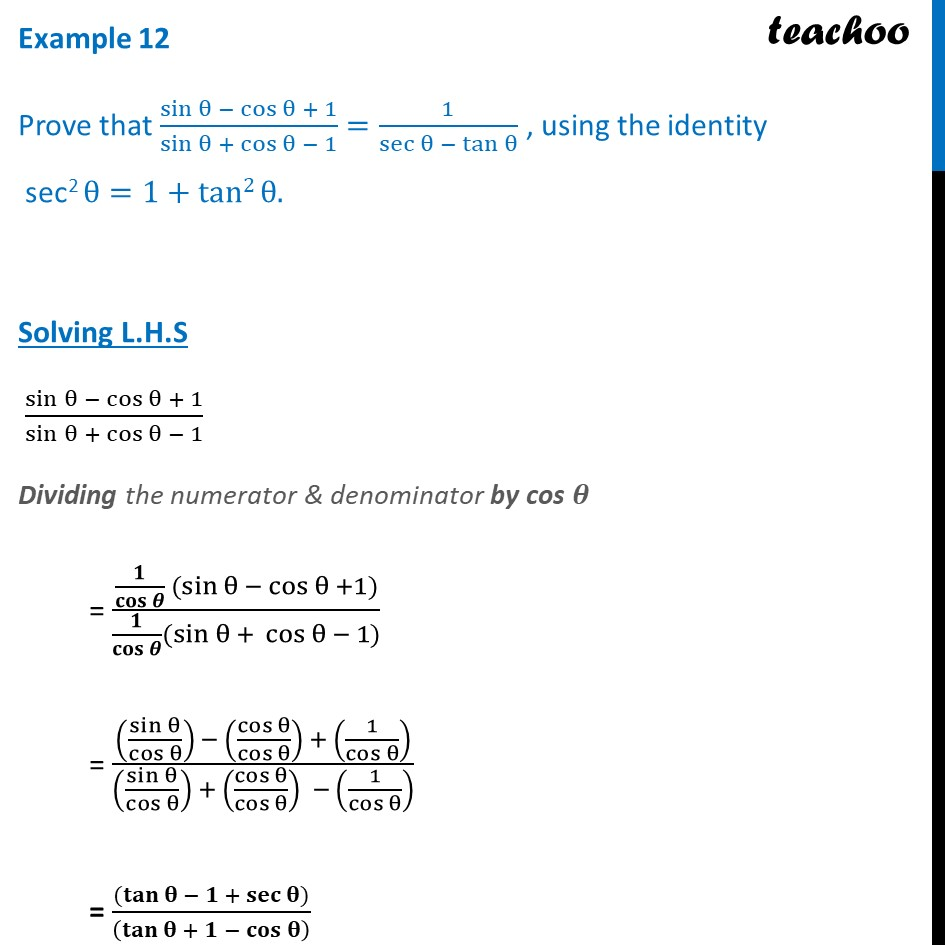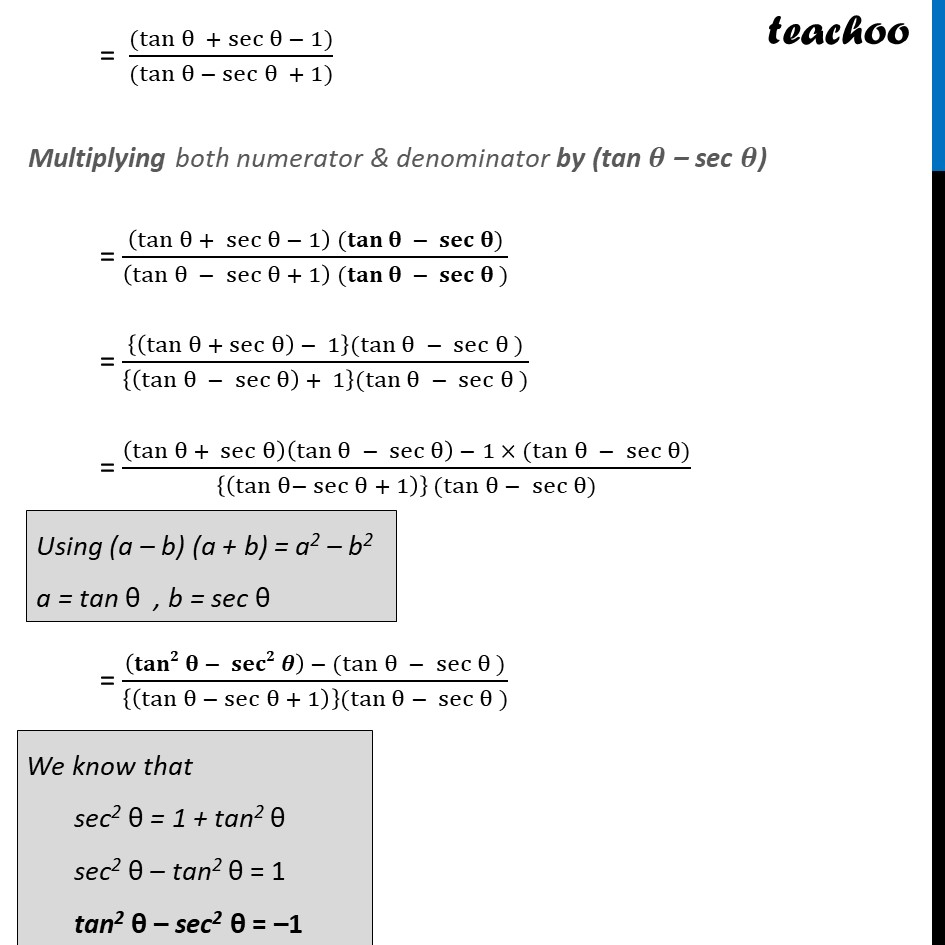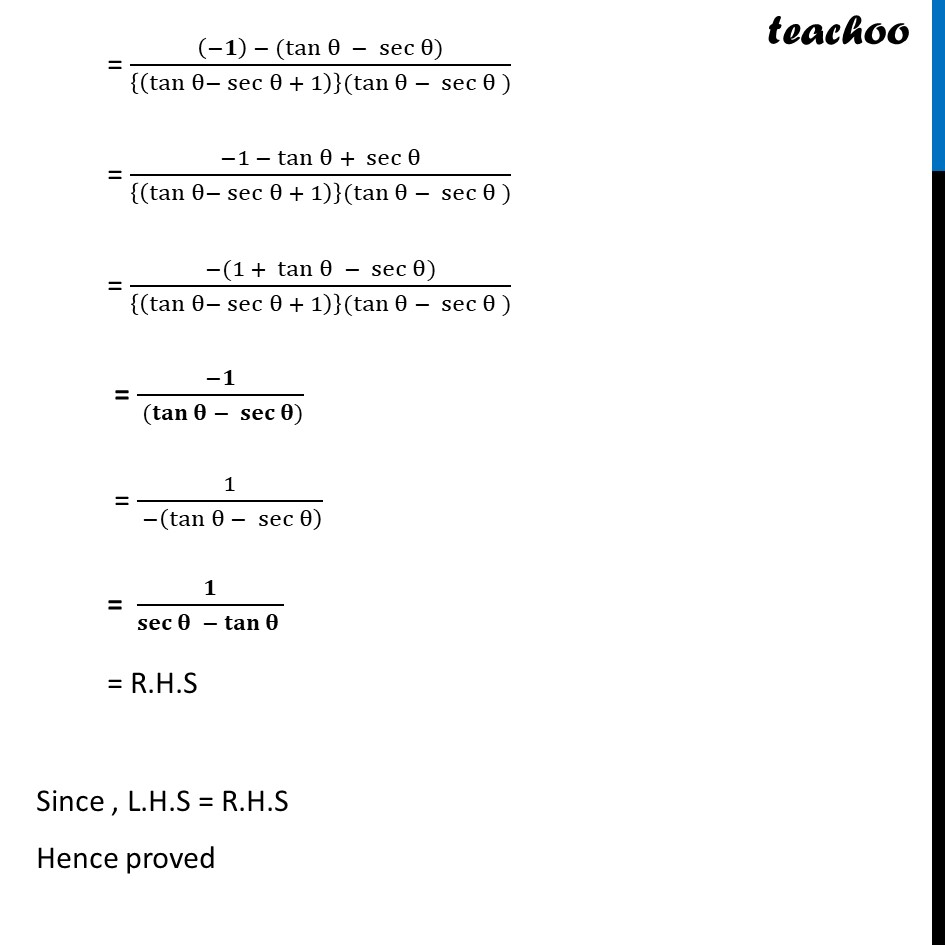Evaluating using Trignometric Identities

Chapter 8 Class 10 Introduction to Trignometry
Concept wiseLearn in your speed, with individual attention - Teachoo Maths 1-on-1 Class

### Transcript

Example 12 Prove that (sin θ − cos θ + 1)/(sin θ + cos θ − 1)=1/(sec θ − tan θ) , using the identity sec2 θ=1+tan2 θ. Solving L.H.S (sin⁡θ − cos θ + 1)/(sin θ + cos θ − 1) Dividing the numerator & denominator by cos 𝜽 = (𝟏/(𝐜𝐨𝐬 𝜽) (sin θ − cos θ +1))/(𝟏/(𝐜𝐨𝐬 𝜽)(sin θ + cos θ − 1)) = (((sin θ)/(cos θ)) − ((cos θ)/(cos θ)) + (1/(cos θ)))/(((sin θ)/(cos θ)) + ((cos θ)/(cos θ)) − (1/(cos θ)) ) = ((𝐭𝐚𝐧 𝛉 − 𝟏 + 𝐬𝐞𝐜 𝛉))/((𝐭𝐚𝐧 𝛉 + 𝟏 − 𝐜𝐨𝐬 𝛉) ) = ((tan θ + sec θ − 1))/((tan θ − sec θ + 1)) Multiplying both numerator & denominator by (tan 𝜽 – sec 𝜽) = ((tan θ + sec θ − 1) (𝐭𝐚𝐧 𝛉 − 𝐬𝐞𝐜 𝛉))/((tan θ − sec θ + 1) (𝐭𝐚𝐧 𝛉 − 𝐬𝐞𝐜 𝛉 )) = ({(tan⁡θ + sec⁡θ ) − 1}(tan θ − sec θ ))/({(tan θ − sec θ) + 1}(tan θ − sec θ )) = ((tan θ + sec θ)(tan θ − sec θ) − 1 × (tan θ − sec θ))/({(tan⁡θ− sec⁡θ + 1)} (tan θ − sec θ)) Using (a – b) (a + b) = a2 – b2 a = tan θ , b = sec θ = ((𝐭𝐚𝐧𝟐 𝛉 − 𝐬𝐞𝐜𝟐 𝜽) − (tan θ − sec θ ))/({(tan⁡θ − sec⁡θ + 1)}(tan θ − sec θ )) We know that sec2 θ = 1 + tan2 θ sec2 θ – tan2 θ = 1 tan2 θ – sec2 θ = –1 = ((−𝟏) − (tan θ − sec θ))/({(tan⁡θ− sec⁡θ + 1)}(tan θ − sec θ )) = (−1 − tan θ + sec θ)/({(tan⁡θ− sec⁡θ + 1)}(tan θ − sec θ )) = (−(1 + tan θ − sec θ))/({(tan⁡θ− sec⁡θ + 1)}(tan θ − sec θ )) = (−𝟏)/( (𝐭𝐚𝐧 𝛉 − 𝐬𝐞𝐜 𝛉)) = 1/( −(tan θ − sec θ) ) = 𝟏/(𝐬𝐞𝐜 𝛉 − 𝐭𝐚𝐧 𝛉 ) = R.H.S Since , L.H.S = R.H.S Hence proved